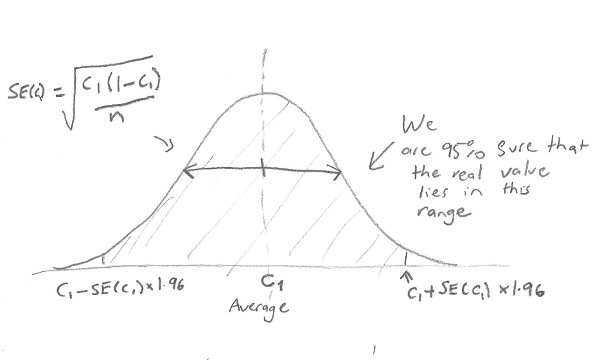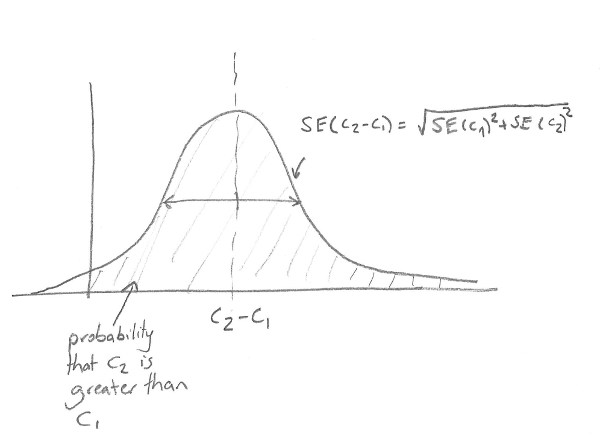# Understanding experiment statistics

The Zeald split testing tool uses industry standard statistics to calculate the winner of a split test, so that you don't need to understand too much about the underlying statistical theory in order to test things on your website.  However, if you like math and you're interested in how it works, read on for a very quick explanation ....

When you perform an experiment a large number of times, as we are doing with the split testing tool, the best estimate you have of the "real" value of the conversion rate of the original page or the alternative page is the average conversion rate.  However this is only an estimate.  In fact, the probability that the real conversion rate takes different values is a normal distribution, a bell shaped curve like below (where c1 = the conversion rate and n=number of visitors).   We can find the probability that the conversion rate lies between any two values by finding the area under this graph:The more times you "run the experiment", ie the more visitors each page in the experiment has, the narrower this curve gets, and the more accurate your idea of c1 is.

So when you run an experiment, and one page has a conversion rate of c1 and the other c2, the probability distributions look like this:Even though the estimate of the conversion rate,  c2, is greater than c1, there's still a lot of overlap and thus a chance that in reality c2 is actually less than c1.   This is why even though the figures are higher for one test or another the split testing report will say that the figures are "inconclusive"

To find out the chance that c2 is really greater than c1, you can calculate the probability distribution of c2-c1, and then calculate the chance that is greater than zero by finding the area under this graph:This probability is the "chance to beat original".  Our split testing software automatically calculates it, and if its greater than 95% for a particular page we tell you that page is a winner.

If you'd like to play with the figures, we've made an excel spreadsheet that calculates these statistics for you.